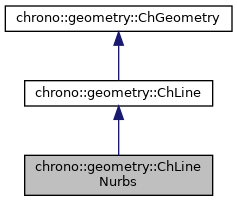chrono::geometry::ChLineNurbs Class Reference

## Description

Geometric object representing a NURBS spline.

#include <ChLineNurbs.h>

Inheritance diagram for chrono::geometry::ChLineNurbs:[legend]
Collaboration diagram for chrono::geometry::ChLineNurbs:[legend]

## Public Member Functions

ChLineNurbs ()
Constructor. By default, a segment (order = 1, two points on X axis, at -1, +1)

ChLineNurbs (int morder, std::vector< ChVector<> > &mpoints, ChVectorDynamic<> *mknots=0, ChVectorDynamic<> *weights=0)
Constructor from a given array of control points. More...

ChLineNurbs (const ChLineNurbs &source)

virtual ChLineNurbsClone () const override
"Virtual" copy constructor (covariant return type).

virtual int Get_complexity () const override
Tell the complexity.

virtual void Evaluate (ChVector<> &pos, const double parU) const override
Evaluates a point on the line, given parametric coordinate U. More...

virtual void Derive (ChVector<> &dir, const double parU) const override
Evaluates a tangent versor, given parametric coordinate. More...

double ComputeUfromKnotU (const double u) const
When using Evaluate() etc. More...

double ComputeKnotUfromU (const double U) const
When using Evaluate() etc. More...

std::vector< ChVector<> > & Points ()
Access the points.

ChVectorDynamicWeights ()
Access the weights.

ChVectorDynamicKnots ()
Access the knots.

int GetOrder ()
Get the order of spline.

virtual void SetupData (int morder, std::vector< ChVector<> > &mpoints, ChVectorDynamic<> *mknots=0, ChVectorDynamic<> *weights=0)
Initial easy setup from a given array of control points. More...

virtual void ArchiveOUT (ChArchiveOut &marchive) override
Method to allow serialization of transient data to archives.

virtual void ArchiveIN (ChArchiveIn &marchive) override
Method to allow de-serialization of transient data from archives.Public Member Functions inherited from chrono::geometry::ChLine
ChLine (const ChLine &source)

virtual GeometryType GetClassType () const override
"Virtual" copy constructor (covariant return type). More...

virtual bool Get_closed () const
Tell if the curve is closed.

virtual void Set_closed (bool mc)

virtual void Set_complexity (int mc)

virtual int GetManifoldDimension () const override
This is a line.

bool FindNearestLinePoint (ChVector<> &point, double &resU, double approxU, double tol) const
Find the parameter resU for the nearest point on curve to "point".

virtual double Length (int sampling) const
Returns curve length. Typical sampling 1..5 (1 already gives correct result with degree1 curves)

virtual ChVector GetEndA () const
Return the start point of the line. More...

virtual ChVector GetEndB () const
Return the end point of the line. More...

double CurveCurveDist (ChLine *compline, int samples) const
Returns adimensional information on "how much" this curve is similar to another in its overall shape (does not matter parametrization or start point). More...

double CurveSegmentDist (ChLine *complinesegm, int samples) const
Same as before, but returns "how near" is complinesegm to whatever segment of this line (does not matter the percentual of line). More...

double CurveCurveDistMax (ChLine *compline, int samples) const
Same as above, but instead of making average of the distances, these functions return the maximum of the distances...

double CurveSegmentDistMax (ChLine *complinesegm, int samples) const

virtual bool DrawPostscript (ChFile_ps *mfle, int markpoints, int bezier_interpolate)
Draw into the current graph viewport of a ChFile_ps file.Public Member Functions inherited from chrono::geometry::ChGeometry
ChGeometry (const ChGeometry &source)

virtual void GetBoundingBox (ChVector<> &cmin, ChVector<> &cmax, const ChMatrix33<> &rot) const
Compute bounding box along the directions defined by the given rotation matrix. More...

void InflateBoundingBox (ChVector<> &cmin, ChVector<> &cmax, const ChMatrix33<> &rot) const
Enlarge the given existing bounding box with the bounding box of this object.

Returns the radius of a bounding sphere for this geometry. More...

virtual ChVector Baricenter () const
Compute center of mass.

virtual void Update ()
Generic update of internal data.

## Public Attributes

std::vector< ChVector<> > points

ChVectorDynamic weights

ChVectorDynamic knots

int pPublic Types inherited from chrono::geometry::ChGeometry
enum  GeometryType {
NONE, SPHERE, BOX, CYLINDER,
TRIANGLE, CAPSULE, CONE, LINE,
LINE_ARC, LINE_BEZIER, LINE_CAM, LINE_PATH,
LINE_POLY, LINE_SEGMENT, ROUNDED_BOX, ROUNDED_CYLINDER,
ROUNDED_CONE, TRIANGLEMESH, TRIANGLEMESH_CONNECTED, TRIANGLEMESH_SOUP
}
Enumeration of geometric objects.Protected Attributes inherited from chrono::geometry::ChLine
bool closed

int complexityU

## ◆ ChLineNurbs()

 chrono::geometry::ChLineNurbs::ChLineNurbs ( int morder, std::vector< ChVector<> > & mpoints, ChVectorDynamic<> * mknots = 0, ChVectorDynamic<> * weights = 0 )

Constructor from a given array of control points.

Input data is copied. If the knots are not provided, a uniformly spaced knot vector is made. If the weights are not provided, a constant weight vector is made.

Parameters
 morder order p: 1= linear, 2=quadratic, etc. mpoints control points, size n. Required: at least n >= p+1 mknots knots, size k. Required k=n+p+1. If not provided, initialized to uniform. weights weights, size w. Required w=n. If not provided, all weights as 1.

## ◆ ComputeKnotUfromU()

 double chrono::geometry::ChLineNurbs::ComputeKnotUfromU ( const double U ) const
inline

When using Evaluate() etc.

you need U parameter to be in 0..1 range, but knot range is not necessarily in 0..1. So you can convert U->u, where u is in knot range, calling this:

## ◆ ComputeUfromKnotU()

 double chrono::geometry::ChLineNurbs::ComputeUfromKnotU ( const double u ) const
inline

When using Evaluate() etc.

you need U parameter to be in 0..1 range, but knot range is not necessarily in 0..1. So you can convert u->U, where u is in knot range, calling this:

## ◆ Derive()

 void chrono::geometry::ChLineNurbs::Derive ( ChVector<> & dir, const double parU ) const
overridevirtual

Evaluates a tangent versor, given parametric coordinate.

Parameter U always work in 0..1 range. Computed value goes into the 'pos' reference.

Reimplemented from chrono::geometry::ChLine.

## ◆ Evaluate()

 void chrono::geometry::ChLineNurbs::Evaluate ( ChVector<> & pos, const double parU ) const
overridevirtual

Evaluates a point on the line, given parametric coordinate U.

Parameter U always work in 0..1 range, even if knots are not in 0..1 range. So if you want to use u' in knot range, use ComputeUfromKnotU(). Computed value goes into the 'pos' reference. It must be implemented by inherited classes.

Implements chrono::geometry::ChLine.

## ◆ SetupData()

 void chrono::geometry::ChLineNurbs::SetupData ( int morder, std::vector< ChVector<> > & mpoints, ChVectorDynamic<> * mknots = 0, ChVectorDynamic<> * weights = 0 )
virtual

Initial easy setup from a given array of control points.

Input data is copied. If the knots are not provided, a uniformly spaced knot vector is made. If the weights are not provided, a constant weight vector is made.

Parameters
 morder order p: 1= linear, 2=quadratic, etc. mpoints control points, size n. Required: at least n >= p+1 mknots knots, size k. Required k=n+p+1. If not provided, initialized to uniform. weights weights, size w. Required w=n. If not provided, all weights as 1.

The documentation for this class was generated from the following files:
• /builds/uwsbel/chrono/src/chrono/geometry/ChLineNurbs.h
• /builds/uwsbel/chrono/src/chrono/geometry/ChLineNurbs.cpp